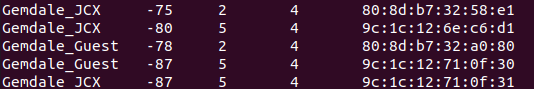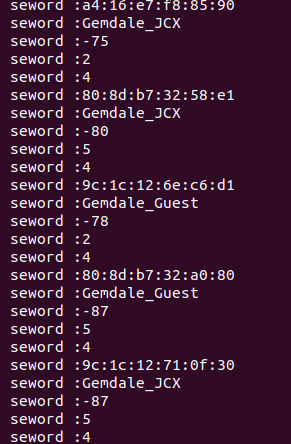前言：int i, j ;    i = 0;    j = strlen(strIn) - 1;    while(strIn[i] == ' ' || strIn[i] == '\t')
++i;    while(strIn[j] == ' ' || strIn[j] == '\t')
--j;
strncpy(strOut, strIn + i , j - i + 1);

解析代码

实际代码如下：

vector<string> Parse_Wifi_List(string& msg){
vector<string> words;        if(msg.empty())            return words;        string::iterator temp_p = msg.begin();        string sepword;
bool bit_true = false;        while(temp_p != msg.end())
{            if(/* * temp_p == ' ' || */  * temp_p == '\t' || * temp_p == '\n')
{                if(bit_true)
{
words.push_back(sepword);
PP_INFO("seword :%s",sepword.c_str());
sepword.clear();
}
bit_true = false;
temp_p++;                continue;
}            else
{
bit_true = true;
sepword += *temp_p;
}            if(*temp_p ++ == '\0')
{                break;
}            // else
// {
//  PP_INFO("%d %d %c",msg.end(),*temp_p,*temp_p);
// }
}        // for(auto point : words)
// {
//  printf(".%s\n",point.c_str());
// }
//for(uint16_t i =0;i<words.size();i+=5)
//{
// printf("[]%s,%s\n",words[i].c_str(),words[i+1].c_str(),words[i+3].c_str());
//}
return words;
}

使用此代码解析LOG如下：结语

‧‧‧‧‧‧‧‧‧‧‧‧‧‧‧‧  END  ‧‧‧‧‧‧‧‧‧‧‧‧‧‧‧‧